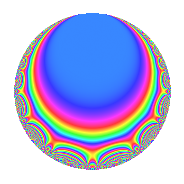# Properties

 Label 6003.2.a.gLevel 6003 Weight 2 Character orbit 6003.a Self dual Yes Analytic conductor 47.934 Analytic rank 0 Dimension 4 CM No Inner twists 1

# Related objects

## Newspace parameters

 Level: $$N$$ = $$6003 = 3^{2} \cdot 23 \cdot 29$$ Weight: $$k$$ = $$2$$ Character orbit: $$[\chi]$$ = 6003.a (trivial)

## Newform invariants

 Self dual: Yes Analytic conductor: $$47.9341963334$$ Analytic rank: $$0$$ Dimension: $$4$$ Coefficient field: 4.4.5744.1 Coefficient ring: $$\Z[a_1, \ldots, a_{17}]$$ Coefficient ring index: $$2$$ Fricke sign: $$-1$$ Sato-Tate group: $\mathrm{SU}(2)$

## $q$-expansion

Coefficients of the $$q$$-expansion are expressed in terms of a basis $$1,\beta_1,\beta_2,\beta_3$$ for the coefficient ring described below. We also show the integral $$q$$-expansion of the trace form.

 $$f(q)$$ $$=$$ $$q -2 q^{4} + \beta_{1} q^{5} + ( -1 - \beta_{2} + \beta_{3} ) q^{7} +O(q^{10})$$ $$q -2 q^{4} + \beta_{1} q^{5} + ( -1 - \beta_{2} + \beta_{3} ) q^{7} + ( 1 - \beta_{1} + \beta_{2} + \beta_{3} ) q^{11} + ( 1 + 2 \beta_{2} ) q^{13} + 4 q^{16} + ( -1 - \beta_{2} + 2 \beta_{3} ) q^{17} + ( 2 + 2 \beta_{2} + \beta_{3} ) q^{19} -2 \beta_{1} q^{20} - q^{23} + ( -2 + \beta_{1} - \beta_{2} + \beta_{3} ) q^{25} + ( 2 + 2 \beta_{2} - 2 \beta_{3} ) q^{28} - q^{29} + ( -1 + 2 \beta_{1} - \beta_{2} + \beta_{3} ) q^{31} + ( -1 + \beta_{1} + \beta_{2} + \beta_{3} ) q^{35} + ( -1 + 3 \beta_{1} - \beta_{2} ) q^{37} + ( -2 + \beta_{1} + 2 \beta_{3} ) q^{41} + ( 1 - 3 \beta_{1} - \beta_{2} ) q^{43} + ( -2 + 2 \beta_{1} - 2 \beta_{2} - 2 \beta_{3} ) q^{44} -\beta_{1} q^{47} + ( 2 - \beta_{1} + \beta_{2} - \beta_{3} ) q^{49} + ( -2 - 4 \beta_{2} ) q^{52} + ( 4 - 2 \beta_{1} + 2 \beta_{2} - 2 \beta_{3} ) q^{53} + ( -2 + 2 \beta_{3} ) q^{55} + ( 6 - 2 \beta_{1} - \beta_{2} + \beta_{3} ) q^{59} + ( -4 - 2 \beta_{1} - 2 \beta_{2} ) q^{61} -8 q^{64} + ( 2 - \beta_{1} - 2 \beta_{2} + 2 \beta_{3} ) q^{65} + ( 2 + 2 \beta_{2} - 2 \beta_{3} ) q^{67} + ( 2 + 2 \beta_{2} - 4 \beta_{3} ) q^{68} + ( 5 + \beta_{1} - 2 \beta_{3} ) q^{71} + ( -5 + \beta_{2} - \beta_{3} ) q^{73} + ( -4 - 4 \beta_{2} - 2 \beta_{3} ) q^{76} + ( -2 + 4 \beta_{1} - 4 \beta_{2} - 2 \beta_{3} ) q^{77} + ( -6 + 2 \beta_{1} + 3 \beta_{3} ) q^{79} + 4 \beta_{1} q^{80} + ( 6 - 3 \beta_{1} + 4 \beta_{2} ) q^{83} + ( -1 + 2 \beta_{1} + \beta_{2} + 3 \beta_{3} ) q^{85} + ( -1 + 6 \beta_{1} - 3 \beta_{2} ) q^{89} + ( -11 + 6 \beta_{1} - 3 \beta_{2} - \beta_{3} ) q^{91} + 2 q^{92} + ( 2 + \beta_{1} - 2 \beta_{2} + 4 \beta_{3} ) q^{95} + ( -4 + 2 \beta_{1} - 2 \beta_{2} ) q^{97} +O(q^{100})$$ $$\operatorname{Tr}(f)(q)$$ $$=$$ $$4q - 8q^{4} + 2q^{5} - 2q^{7} + O(q^{10})$$ $$4q - 8q^{4} + 2q^{5} - 2q^{7} + 16q^{16} - 2q^{17} + 4q^{19} - 4q^{20} - 4q^{23} - 4q^{25} + 4q^{28} - 4q^{29} + 2q^{31} - 4q^{35} + 4q^{37} - 6q^{41} - 2q^{47} + 4q^{49} + 8q^{53} - 8q^{55} + 22q^{59} - 16q^{61} - 32q^{64} + 10q^{65} + 4q^{67} + 4q^{68} + 22q^{71} - 22q^{73} - 8q^{76} + 8q^{77} - 20q^{79} + 8q^{80} + 10q^{83} - 2q^{85} + 14q^{89} - 26q^{91} + 8q^{92} + 14q^{95} - 8q^{97} + O(q^{100})$$

Basis of coefficient ring in terms of a root $$\nu$$ of $$x^{4} - 5 x^{2} - 2 x + 1$$:

 $$\beta_{0}$$ $$=$$ $$1$$ $$\beta_{1}$$ $$=$$ $$\nu^{3} - 4 \nu - 1$$ $$\beta_{2}$$ $$=$$ $$\nu^{2} - \nu - 3$$ $$\beta_{3}$$ $$=$$ $$-\nu^{3} + \nu^{2} + 5 \nu - 1$$
 $$1$$ $$=$$ $$\beta_0$$ $$\nu$$ $$=$$ $$($$$$\beta_{3} - \beta_{2} + \beta_{1} - 1$$$$)/2$$ $$\nu^{2}$$ $$=$$ $$($$$$\beta_{3} + \beta_{2} + \beta_{1} + 5$$$$)/2$$ $$\nu^{3}$$ $$=$$ $$2 \beta_{3} - 2 \beta_{2} + 3 \beta_{1} - 1$$

## Embeddings

For each embedding $$\iota_m$$ of the coefficient field, the values $$\iota_m(a_n)$$ are shown below.

For more information on an embedded modular form you can click on its label.

Label $$\iota_m(\nu)$$ $$a_{2}$$ $$a_{3}$$ $$a_{4}$$ $$a_{5}$$ $$a_{6}$$ $$a_{7}$$ $$a_{8}$$ $$a_{9}$$ $$a_{10}$$
1.1
 0.291367 −1.92022 −0.751024 2.37988
0 0 −2.00000 −2.14073 0 2.72347 0 0 0
1.2 0 0 −2.00000 −0.399447 0 −3.44099 0 0 0
1.3 0 0 −2.00000 1.58049 0 −3.08254 0 0 0
1.4 0 0 −2.00000 2.95969 0 1.80007 0 0 0
 $$n$$: e.g. 2-40 or 990-1000 Significant digits: Format: Complex embeddings Normalized embeddings Satake parameters Satake angles

## Inner twists

This newform does not admit any (nontrivial) inner twists.

## Atkin-Lehner signs

$$p$$ Sign
$$3$$ $$-1$$
$$23$$ $$1$$
$$29$$ $$1$$

## Hecke kernels

This newform can be constructed as the intersection of the kernels of the following linear operators acting on $$S_{2}^{\mathrm{new}}(\Gamma_0(6003))$$:

 $$T_{2}$$ $$T_{5}^{4} - 2 T_{5}^{3} - 6 T_{5}^{2} + 8 T_{5} + 4$$